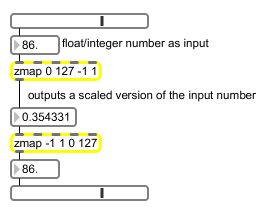# zmap

Maps input range of values to output range

## Description

zmap will take a specified input range of values and map them to an output range of values.

## Arguments

Name Type Opt Description
minimum-input maximum-input minimum-output and maximum-output int or float opt The first argument is the minimum input value, the second argument is the maximum input value. The third and fourth arguments are the minimum and maximum output values, respectively. If either of the low values is higher than the corresponding high value (or vice versa), the two values are reversed to preserve the high-low relationship. Note that this is different from the behavior of the scale object, which lets you do reverse scaling. Also, note that the zmap object will clip to the boundaries of the output range.

## Messages

 int input [int] Converted to float. float input [float] In left inlet: The incoming value is scaled according to the mapping provided by the arguments, or values received in the other inlets. Note: The preservation of the high-low relationship is different from the behavior of the scale object, which lets you do reverse scaling. Also, note that the zmap object will clip to the boundaries of the output range. (inlet1) low-input-value [float] In second inlet: Sets the low input value. If the value is higher than the high input value, the two values are reversed to preserve the high-low relationship. (inlet2) high-input-value [float] In third inlet: Sets the high input value. If the value is lower than the low input value, the two values are reversed to preserve the high-low relationship. (inlet3) low-output-value [float] In fourth inlet: Sets the low output value. If the value is higher than the high output value, the two values are reversed to preserve the high-low relationship. (inlet4) high-output-value [float] In right inlet: Sets the high output value. If the value is higher than the high output value, the two values are reversed to preserve the high-low relationship. list input [list] In left inlet: A list of numbers sent to the left inlet will trigger the output of a list consisting of the results of performing the specified scaling operation on each item in the input list.

## Output

float: When zmap receives a value in its leftmost inlet, that value is scaled to the indicated output range of values.

## ExamplesAn example of how to map an integer slider into a useful range of floating-point values and back again